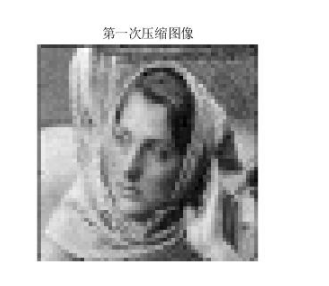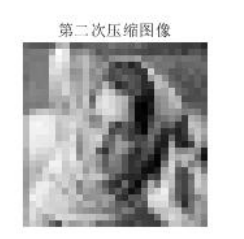• ###### MATLAB图像处理

全面系统的学习MATLAB在图像处理中的应用

19908 人正在学习 去看看 魏伟

http://media.cs.tsinghua.edu.cn/~ahz/digitalimageprocess/chapter12/chapt12_ahz.htm

Matlab 小波变换

lean图像的行列应该满足2的幂次方

img  =  imread('lena.jpg');
img =rgb2gray(img);
img = double(img);

[ca1 ch1,cv1,cd1] = dwt2(img,'haar');
figure;
imshow([uint8(ca1),uint8(ch1);uint8(cv1),uint8(cd1)]);
title('first wavelet decomposition');

[ca2 ch2,cv2,cd2] = dwt2(ca1,'haar');
figure;
imshow([uint8(ca2),uint8(ch2);uint8(cv2),uint8(cd2)]);
title('second wavelet decomposition');

% restruct img
img_ca1 =  idwt2(ca2,ch2,cv2,cd2,'haar');
img_re = idwt2(img_ca1,ch1,cv1,cd1,'haar');
figure;
imshow( uint8(img_re));
title('wavelet restruct');

2017-10-24 16:15:56 HelloZEX 阅读数 9199
• ###### MATLAB图像处理

全面系统的学习MATLAB在图像处理中的应用

19908 人正在学习 去看看 魏伟

# 小波理论的基本概念及概述（第二版）## 变换…啥？## 为什么需要频率信息？## 终极解决方案：小波变换http://blog.csdn.net/alihouzi/article/category/3132373

2014-10-26 21:53:38 EbowTang 阅读数 14952
• ###### MATLAB图像处理

全面系统的学习MATLAB在图像处理中的应用

19908 人正在学习 去看看 魏伟

# 一，小波阈值去噪基本理论

图像在获取或传输过程中会因各种噪声的干扰使质量下降,这将对后续图像的处理产生不利影响.所以必须对图像进行去噪处理,而去噪所要达到的目的就是在较好去除噪声的基础上,良好的保持图像的边缘等重要细节.在图像去噪领域得到广泛的应用.本博文根据小波的分解与重构原理，实现了基于硬阈值和软阈值函数的小波阈值去噪的C++版本，最终结果与matlab库函数运算结果完全一致。

## 1，小波阈值处理

【1】 Donoho D L. De-noising by soft-thresholding. IEEE Trans- actions on Information Theory, 1995, 41(3): 613−627
【2】 Donoho D L, Johnstone I M. Adapting to unknown smooth- ness via wavelet shrinkage. Journal of the American Statistic
Association, 1995, 90(432): 1200−1224
【3】 Donoho D L, Johnstone I M, Kerkyacharian G, Picard D. Wavelet shrinkage: asymptopia? Journal of Royal Statisti-
cal Society Series B, 1995, 57(2): 301−369

2，阈值函数的选取

阈值去噪中,阈值函数体现了对超过和低于阈值的小波系数不同处理策略，是阈值去噪中关键的一步。设w表示小波系数,T为给定阈值,sign(*)为符号函数,常见的阈值函数有：1) 硬阈值函数在阈值点是不连续的，在下图中已经用黑线标出。不连续会带来振铃，伪吉布斯效应等。

2) 软阈值函数，原系数和分解得到的小波系数总存在着恒定的偏差,这将影响重构的精度使得重构图像的边缘模糊等现象.## 4，阈值策略## 5，小波阈值去噪过程

有用信号经小波变换后, 其能量将集中在少数的小波系数上, 而噪声点的小波系数互不相关, 分布在各个尺度的所有时间轴上. 保留小波变换的各尺度下的模极大值点, 而将其他点置零或最大程度的减小, 然后将处理后的小波系数做小波逆变换, 即可达到抑制噪声的目的.阈值去噪是通过对变换域系数与阈值进行比较判断, 然后将处理后的系数进行逆变换重构去噪图像. 小波阈值去噪法的具体步骤如下:

信号和噪声在小波域内具有不同的相关性. 信号在尺度间相应位置上的小波系数具有很强的相关性, 而噪声的小波系数则具有弱相关性或者不相关. 在阈值去噪中, 由于所选定的阈值通常固定, 不会随着小波系数的不同而变化, 这就不可避免地会对部分小波系数进行误判，于是又养活了一批学者。。。分析改进，分析改进，发文章！1），小波的分解2，小波的重构# 二，Matlab库函数实现

## 1，核心库函数说明

1）wavedec2

2）waverec2

3）wthresh函数

clear;
y = linspace(-1,1,100);
thr = 0.4;
ythard = wthresh(y,'h',thr);
ytsoft = wthresh(y,'s',thr);
subplot(311);plot(y);
subplot(312);plot(ythard);
subplot(313);plot(ytsoft);## 2，软，硬阈值处理效果：## 3，完整的代码实现

% 获取输入参数
w    = 'db3';%小波类型
n    = 3;%分解层数

sorh1 = 'hard';%硬阈值
sorh2 = 'soft';%软阈值
sorh3 = 'half';%半阈值
% 对图像进行小波分解
[c,l] = wavedec2(octimage,n,w);

%求取阈值
N = numel(octimage);
[chd1,cvd1,cdd1] = detcoef2('all',c,l,1);
cdd1=cdd1(:)';
sigma = median(abs(cdd1))/0.6745;%提取细节系数求中值并除以0.6745
thr = sigma*sqrt(2*log(N))/sqrt(1+sqrt(n)); %对阈值做了改进

% 对小波系数全局阈值处理
cxchard = c;% 保留近似系数
cxcsoft = c;% 保留近似系数
cxchalf = c;% 保留近似系数
justdet = prod(l(1,:))+1:length(c);%截取细节系数（不处理近似系数）

% 阈值处理细节系数
cxchard(justdet) = myWthresh(cxchard(justdet),sorh1,thr);%硬阈值去噪
cxcsoft(justdet) = myWthresh(cxcsoft(justdet),sorh2,thr);%软阈值去噪
cxchalf(justdet) = myWthresh(cxchalf(justdet),sorh3,thr);%软阈值去噪

%小波重建
xchard = waverec2(cxchard,l,w);
xcsoft = waverec2(cxcsoft,l,w);
xchalf = waverec2(cxchalf,l,w);

figure(2);
imshow(uint8(xchard(1:iCount, :)));title('硬阈值去噪图像');

figure(3);
imshow(uint8(xcsoft(1:iCount, :)));title('软阈值去噪图像');

figure(4);
imshow(uint8(xchalf(1:iCount, :)));title('半阈值去噪图像'); 

# 三，C加加实现

//初始化二维图像的分解信息，保存未来需要的信息
bool  CWavelet::InitDecInfo2D(
const int height,//预分解的图像的高度
const int width,//预分解的图像的宽度
const int Scale,//分解尺度
const int dbn//db滤波器编号，有默认值
)
{
if (dbn != 3)
SetFilter(dbn);

if (height < m_dbFilter.filterLen - 1 || width < m_dbFilter.filterLen - 1)
{
cerr << "错误信息：滤波器长度大于信号的高度或者宽度!" << endl;
return false;
}

int srcHeight = height;
int srcWidth = width;
m_msgCL2D.dbn = dbn;
m_msgCL2D.Scale = Scale;
m_msgCL2D.msgHeight.resize(Scale + 2);
m_msgCL2D.msgWidth.resize(Scale + 2);
//源图像的尺寸
m_msgCL2D.msgHeight = height;
m_msgCL2D.msgWidth = width;

//每一尺度上的尺寸
for (int i = 1; i <= Scale; i++)//注意：每个尺度的四个分量的长宽是一样的
{
int exHeight = (srcHeight + m_dbFilter.filterLen - 1) / 2;//对称拓延后系数的长度的一半
srcHeight = exHeight;
m_msgCL2D.msgHeight[i] = srcHeight;

int exWidth = (srcWidth + m_dbFilter.filterLen - 1) / 2;//对称拓延后系数的长度一半
srcWidth = exWidth;
m_msgCL2D.msgWidth[i] = srcWidth;
}
m_msgCL2D.msgHeight[Scale + 1] = srcHeight;
m_msgCL2D.msgWidth[Scale + 1] = srcWidth;

//计算总的数据个数
int tmpAllSize = 0;
int curPartSize = 0;
int prePartSize = 0;
for (int i = 1; i <= Scale; i++)
{
curPartSize = m_msgCL2D.msgHeight[i] * m_msgCL2D.msgWidth[i];
tmpAllSize += curPartSize * 4 - prePartSize;
prePartSize = curPartSize;
}
m_msgCL2D.allSize = tmpAllSize;
m_bInitFlag2D = true;
return true;
}

## 1，核心函数的实现

### 1）二维信号的单次分解

// 二维数据的小波分解
void  CWavelet::DWT2(
double *pSrcImage,//源图像数据(存储成一维数据，行优先存储)
int height,//图像的高
int width,//图像的宽
double *pDstCeof//分解出来的图像系数
)
{

if (!m_bInitFlag2D)
{
cerr << "错误信息：未初始化，无法对信号进行分解!" << endl;
return;
}

if (pSrcImage == NULL || pDstCeof == NULL)
{
cerr << "错误信息：dwt2数据无内存" << endl;
Sleep(3000);
exit(1);
}

int exwidth = (width + m_dbFilter.filterLen - 1) / 2 * 2;//pImagCeof的宽度
int exheight = (height + m_dbFilter.filterLen - 1) / 2 * 2;//pImagCeof的高度

double *tempImage = new double[exwidth*height];

// 对每一行进行行变换
double *tempAhang = new double[width];
double *tempExhang = new double[exwidth]; // 临时存放每一行的处理数据
for (int i = 0; i < height; i++)
{
for (int j = 0; j < width; j++)
tempAhang[j] = pSrcImage[i*width + j];//提取每一行的数据

DWT(tempAhang, width, tempExhang);

for (int j = 0; j < exwidth; j++)
tempImage[i*exwidth + j] = tempExhang[j];
}

// 对每一列进行列变换
double *tempAlie = new double[height]; // 临时存放每一列的转置数据
double *tempexlie = new double[exheight]; // 临时存放每一列的处理数据
for (int i = 0; i < exwidth; i++)
{
// 列转置
for (int j = 0; j < height; j++)
tempAlie[j] = tempImage[j*exwidth + i];//提取每一列数据

//执行变换
DWT(tempAlie, height, tempexlie);

// 反转置
for (int j = 0; j < exheight; j++)
pDstCeof[j*exwidth + i] = tempexlie[j];
}

delete[] tempAlie;
tempAlie = NULL;
delete[] tempexlie;
tempexlie = NULL;

delete[] tempAhang;
tempAhang = NULL;
delete[] tempExhang;
tempExhang = NULL;

delete[] tempImage;
tempImage = NULL;

}

### 2）二维信号的单次重构

//二维小波反变换
void  CWavelet::IDWT2(
double *pSrcCeof, //二维源图像系数数据
int dstHeight,//重构出来后数据的高度
int dstWidth,//重构出来后数据的宽度
double *pDstImage//重构出来的图像
)
{
int srcHeight = (dstHeight + m_dbFilter.filterLen - 1) / 2 * 2;
int srcWidth = (dstWidth + m_dbFilter.filterLen - 1) / 2 * 2;//pSrcCeof的高度

double *tempAline = new double[srcHeight]; // 临时存放每一列的数据
double *tempdstline = new double[dstHeight]; // 临时存放每一列的重构结果

double *pTmpImage = new double[srcWidth*dstHeight];
// 列重构
for (int i = 0; i < srcWidth; i++)//每一列
{
// 列转置
for (int j = 0; j<srcHeight; j++)
tempAline[j] = pSrcCeof[j*srcWidth + i];//提取每一列

IDWT(tempAline, dstHeight, tempdstline);

// 反转置
for (int j = 0; j < dstHeight; j++)
pTmpImage[j*srcWidth + i] = tempdstline[j];
}

// 对每一行进行行变换
double *tempAhang = new double[srcWidth];
double *tempdsthang = new double[dstWidth]; // 临时存放每一行的处理数据
for (int i = 0; i < dstHeight; i++)
{
for (int j = 0; j < srcWidth; j++)
tempAhang[j] = pTmpImage[i*srcWidth + j];//提取每一行的数据

IDWT(tempAhang, dstWidth, tempdsthang);

for (int j = 0; j < dstWidth; j++)
pDstImage[i*dstWidth + j] = tempdsthang[j];
}

delete[] tempAline;
tempAline = NULL;
delete[] tempdstline;
tempdstline = NULL;

delete[] tempAhang;
tempAhang = NULL;
delete[] tempdsthang;
tempdsthang = NULL;

delete[] pTmpImage;
pTmpImage = NULL;
}

### 3）二维信号的多级分解

// 二维小波多级分解，需要先初始化获取未来数据信息
bool CWavelet::WaveDec2(
double *pSrcData,//源图像数据，存储为一维信号
double *pDstCeof//分解后的系数，它的大小必须是m_msgCL2D.allSize
)
{
if (!m_bInitFlag2D)
{
cerr << "错误信息：未初始化，无法对图像进行分解!" << endl;
return false;
}
if (pSrcData == NULL || pDstCeof == NULL)//错误：无内存
return false;

int height = m_msgCL2D.msgHeight;
int width = m_msgCL2D.msgWidth;
int scale = m_msgCL2D.Scale;

// 临时变量，图像数据
double *tempImage = new double[height*width];
int maxCoefSize =4 * m_msgCL2D.msgHeight * m_msgCL2D.msgWidth;
double *tempDst = new double[maxCoefSize];

for (int i = 0; i < height*width; i++)
tempImage[i] = pSrcData[i];

int gap = m_msgCL2D.allSize - maxCoefSize;
for (int i = 1; i <= scale; i++)
{
DWT2(tempImage, height, width, tempDst);

// 低频子图像的高和宽
height = m_msgCL2D.msgHeight[i];
width = m_msgCL2D.msgWidth[i];

for (int j = 0; j < height*width; j++)
tempImage[j] = tempDst[j];//提取低频系数（近似系数）
//
for (int j = 0, k = gap; j < 4 * height*width; j++, k++)
pDstCeof[k] = tempDst[j];//所有系数
gap -= 4 * m_msgCL2D.msgWidth[i + 1] * m_msgCL2D.msgHeight[i + 1] - height*width;
}
delete[] tempDst;
tempDst = NULL;
delete[] tempImage;
tempImage = NULL;
return true;
}

### 4）多级分解系数的重构

// 根据多级分解系数重构出二维信号，必须先初始化获取分解信息
bool CWavelet::WaveRec2(
double *pSrcCoef,//多级分解出的源系数
double *pDstData//重构出来的信号
)
{
if (!m_bInitFlag2D)
{
cerr << "错误信息：未初始化，无法对信号进行分解!" << endl;
return false;
}
if (pSrcCoef == NULL || pDstData == NULL)//错误：无内存
return false;

int height = m_msgCL2D.msgHeight;
int width = m_msgCL2D.msgWidth;
int decLevel = m_msgCL2D.Scale;

int maxCeofSize = 4 * m_msgCL2D.msgHeight * m_msgCL2D.msgWidth;

double *pTmpImage = new double[maxCeofSize];

int minCeofSize = 4 * m_msgCL2D.msgHeight[decLevel] * m_msgCL2D.msgWidth[decLevel];
for (int i = 0; i < minCeofSize; i++)
pTmpImage[i] = pSrcCoef[i];

int gap = minCeofSize;
for (int i = decLevel; i >= 1; i--)
{
int nextheight = m_msgCL2D.msgHeight[i - 1];//重构出来的高度
int nextwidth = m_msgCL2D.msgWidth[i - 1];//重构出来的宽度
IDWT2(pTmpImage, nextheight, nextwidth, pDstData);

if (i > 1)//i==1已经重构出来了，不再需要提取系数
{
for (int j = 0; j < nextheight*nextwidth; j++)
pTmpImage[j] = pDstData[j];
for (int j = 0; j < 3 * nextheight*nextwidth; j++)
pTmpImage[nextheight*nextwidth + j] = pSrcCoef[gap + j];
gap += 3 * nextheight*nextwidth;
}
}

delete[] pTmpImage;
pTmpImage = NULL;

return true;
}

## 2，函数正确性验证

### 1），二维单次分解与重构测试### 2）二维多级分解与重构测试## 3，阈值去噪结果：

### 硬阈值去噪结果### 软阈值去噪结果clc;
clear all;
close all;
% %%%%%%%%%%%%%%%%%%%%%%%%通过matlab的函数来实现阈值去噪%%%%%%%%%%%%%%%%%%%%%%%%%% %
X=[ 10, 12, 30, 4, 5, 61, 2, 3;
41, 5, 6, 27, 3, 4, 15, 6;
72, 8, 41, 5, 6, 7, 8, 9;
5, 64, 7, 8, 9, 14, 6, 27;
8, 9, 40, 31,10, 12, 30, 4;
50, 61, 2, 3, 41, 5, 6, 27];

X=double(X);

% 获取输入参数
wname    = 'db3';%小波类型
n    = 3;%分解层数
sorh1 = 'h';%硬阈值
sorh2 = 's';%软阈值

% 对图像进行小波分解
[c,l] = wavedec2(X,n,wname);

%求取阈值
N = numel(X);
[chd1,cvd1,cdd1] = detcoef2('all',c,l,1);
cvd1=cvd1(:)';
sigma = median(abs(cvd1))/0.6745;%提取细节系数求中值并除以0.6745
thr = sigma*sqrt(2*log(N));

% 对小波系数全局阈值处理
cxch = c;% 保留近似系数
cxcs = c;% 保留近似系数
justdet = prod(l(1,:))+1:length(c);%截取细节系数（不处理近似系数）

% 阈值处理细节系数
cxch(justdet) = wthresh(cxch(justdet),sorh1,thr);%硬阈值去噪
cxcs(justdet) = wthresh(cxcs(justdet),sorh2,thr);%软阈值去噪

%小波重建
xch = waverec2(cxch,l,wname);
xcs = waverec2(cxcs,l,wname);  

1，对程序要有自己的思考，要有自己的验证和学习过程，写时当时我还是个C++新手！点击下载完整程序
2，二维小波变换不建议用，因为速度太慢了（自己徒手写的），一维可以用。
3，推荐你另外的小波库网站，http://wavelet2d.sourceforge.net/

# 参考资源：

【1】《数字图像处理》（冈萨雷斯matlab第二版）
【2】 Donoho D L. De-noising by soft-thresholding. IEEE Trans- actions on Information Theory, 1995, 41(3): 613−627
【3】 Donoho D L, Johnstone I M. Adapting to unknown smooth- ness via wavelet shrinkage. Journal of the American Statistic
Association, 1995, 90(432): 1200−1224
【4】 Donoho D L, Johnstone I M, Kerkyacharian G, Picard D. Wavelet shrinkage: asymptopia? Journal of Royal Statisti-
cal Society Series B, 1995, 57(2): 301−369
【5】杨恢先，王绪四，改进阈值与尺度间相关的小波红外图像去噪
【6】《小波分析及其应用》，孙延奎著
【7】杨建国.小波分析及其工程应用[M].北京：机械工业出版社.2005
【8】毛艳辉.小波去噪在语音识别预处理中的应用.上海交通大学硕士学位论文.2010
【9】matlab各种函数说明，及其内部函数实现
【10】http://www.bearcave.com/misl/misl_tech/wavelets/haar.html

2019-10-13 21:00:10 weixin_44225182 阅读数 197
• ###### MATLAB图像处理

全面系统的学习MATLAB在图像处理中的应用

19908 人正在学习 去看看 魏伟

## 小波变换实现图像压缩

X=imread('a5.jpg');
X=rgb2gray(X);
subplot(221); imshow(X);
title('原始图像');
%对图像用小波进行层小波分解
[c,s]=wavedec2(X,2,'haar');
%提取小波分解结构中的一层的低频系数和高频系数
cal=appcoef2(c,s,'haar',1);
ch1=detcoef2('h',c,s,1);      %水平方向
cv1=detcoef2('v',c,s,1);      %垂直方向
cd1=detcoef2('d',c,s,1);      %斜线方向
%各频率成份重构
a1=wrcoef2('a',c,s,'haar',1);
h1=wrcoef2('h',c,s,'haar',1);
v1=wrcoef2('v',c,s,'haar',1);
d1=wrcoef2('d',c,s,'haar',1);
c1=[a1,h1;v1,d1];
subplot(222),imshow(c1,[]);
title ('分解后低频和高频信息');
%进行图像压缩
%保留小波分解第一层低频信息
%首先对第一层信息进行量化编码
ca1=appcoef2(c,s,'haar',1);
ca1=wcodemat(ca1,440,'mat',0);
%改变图像高度并显示
ca1=0.5*ca1;
subplot(223);imshow(cal,[]);
title('第一次压缩图像');
%保留小波分解第二层低频信息进行压缩
ca2=appcoef2(c,s,'haar',2);
%首先对第二层信息进行量化编码
ca2=wcodemat(ca2,440,'mat',0);
%改变图像高度并显示
ca2=0.25*ca2;
subplot(224);imshow(ca2,[]);
title('第二次压缩图像');2016-11-07 10:56:25 shenziheng1 阅读数 3861
• ###### MATLAB图像处理

全面系统的学习MATLAB在图像处理中的应用

19908 人正在学习 去看看 魏伟

# 2.小波图像去燥实现的步骤：

1.二维信号的小波分解。选择一个小波和小波分解的层次N，然后计算信号s到第N层的分解。

2.对高频系数进行阈值量化，对于从1~N的每一层，选择一个阈值，并对这一层的高频系数进行软阈值量化处理。

3.二维小波重构

# 3.小波系数阈值降噪

<span style="font-size:18px;">clear all;
subplot(221);image(X);
colormap(map);
xlabel('(a)原始图像');
axis square
%产生含噪声图像
init=2055615866;randn('seed',init)
x=X+50*randn(size(X));
subplot(222);image(x);
colormap(map);
xlabel('(b)含噪声图像');
axis square
%下面进行图像的去噪处理
%用小波画数coif3对x进行2层小波分解
[c,s]=wavedec2(x,2,'coif3');
%提取小波分解中第一层的低频图像，即实现了低通滤波去噪
%设置尺度向量n
n=[1,2];
%设置阈值向量p
p=[10.12,23.28];
%对三个方向高频系数进行阈值处理
nc=wthcoef2('h',c,s,n,p,'s');
nc=wthcoef2('v',c,s,n,p,'s');
nc=wthcoef2('d',c,s,n,p,'s');
%对新的小波分解结构[nc，s]进行重构
x1=waverec2(nc,s,'coif3');
subplot(223);image(x1);
colormap(map);
xlabel('(c)第一次去噪后的图像');
axis square;
xx=wthcoef2('v',nc,s,n,p,'s');
x2=waverec2(xx,s,'coif2');%图像的二维小波重构
subplot(2,2,4);image(x2);
colormap(map);
xlabel('(d)第二次消噪后图解');
axis square;
</span># 4.全局软阈值降噪

<span style="font-size:18px;"><span style="font-size:18px;">clear all;
subplot(131);image(X);
colormap(map);
xlabel('(a)原始图像');
axis square;
init=255615866;
randn('state',init);  %添加随机值
x=X+20*randn(size(X));
subplot(132);image(x);
colormap(map);
xlabel('(b)含噪图像');
axis square;
[thr,sorh,kep]=ddencmp('den','wv',x);   %使用全局阈值选项进行图像消噪处理
xd=wdencmp('gbl',x,'sym5',2,thr,sorh,kep);
subplot(133);image(xd)
colormap(map);
xlabel('(c)消噪图像');
axis square;</span><span style="font-size:24px;">
</span></span>